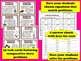# Comparison Conundrums math story problems task cards + printables (set a)Subject
Grade Levels
Resource Type
Product Rating
File Type

PDF (Acrobat) Document File

Be sure that you have an application to open this file type before downloading and/or purchasing.

4 MB|15 pages
Share
Product Description
Build your students’ understanding of multiplicative and additive comparisons with this set of task cards and printables. The 32 task cards in this set will allow your students to practice distinguishing between story problems that are best represented with multiplication & division equations and ones that show the characteristics of addition & subtraction. Extend your students’ practice (or assess their mastery of interpreting comparative situations) with the included activity sheet. With these resources, your students will grow stronger in their understanding of a key concept that dominates the Grade 4 Common Core Math Standards for operations & algebraic thinking and lays the foundation for proportional reasoning.

NOTE: This set of resources is available in a money-saving bundle with three other products that focus on comparative reasoning. The bundle contains three different sets of task cards and printables, a set of five I Have…Who Has? and accompanying printables, and 2 bonus sets of I Have…Who Has? cards that are only available in the bundle. Purchase the bundle here and save 20% off the cost of the individual products!

_____________________________________________________________________________

Common Core State Standards for Mathematics addressed:
Operations & Algebraic Thinking (4.OA)
• Interpret a multiplication equation as a comparison, e.g., interpret 35= 5 × 7 as a statement that 35 is 5 times as many as 7 and 7 times as many as 5. Represent verbal statements of multiplicative comparisons as multiplication equations. (4.OA.1)
• Multiply or divide to solve word problems involving multiplicative comparison, e.g., by using drawings and equations with a symbol for the unknown number to represent the problem, distinguishing multiplicative comparison from additive comparison. (4.OA.2)
_____________________________________________________________________________

Included:
• 32 task cards
• graphic reference sheet
• 2 different answer sheets (keys included for both)
• two-sided activity sheet (scoring guide included)

The printables consist of a graphic reference sheet and a two-page activity sheet that can be used to evaluate student understanding of interpreting story problems. The reference sheet describes the difference between additive and multiplicative comparisons (though it does not use those exact terms as they are not exactly kid-friendly!), using graphics to illustrate a situation in which addition/subtraction would be used to compare and a different situation in which multiplication/division would be used. You can give this to the students before they work on the cards and allow the students to use the sheet as a reference while they complete the cards. Have the students glue it across two pages in their journal, or fold it in half and glue down on one page, allowing them to fold it open and read it.

There is one set of 32 task cards and two different answer sheets & keys, allowing the single set of task cards to do double duty within your class. Each of the 32 cards presents the students with a story problem that involves a comparison. Some of the comparisons are multiplicative (ex: One bird flies at 18 mph, which is 3 times faster than another bird. How fast does the second bird fly?) and others are additive (ex: One bird flies at 24 mph, which is 6 mph faster than another bird. How fast does the second bird fly?).

Of the two answer sheets, the first one presents students with six equations, each using a question mark in place of the unknown. The students are asked to identify any and all equations that match the story problem on the card. In some cases, only one equation matches the given story, and in others as many as four equations match the story. This answer sheet focuses less on solving the problem than on thinking about ways in which it can be represented with multiplication, division, addition, or subtraction. Since there is not a set # of correct equations for each card, students will have to think carefully about the structure of the problem and also about the relationship between multiplication & division and addition & subtraction. The other answer sheet is more straightforward, simply asking the students to solve the problem on each card. You can use either or both of the answer sheets, depending on what skill you would like to emphasize. Your students may use the task cards initially to identify the equations that match the story, and then later use the same cards again to find the answer.

Once students have completed the task cards, you can give them the worksheet to assess their ability to interpret story problems, identify equations that match story situations, and write and solve their own equations that match given story problems. The worksheet has a scoring guide that spells out how many points each part of the worksheet is worth, with each worksheet having a total of 20 points for easier calculation of a percent grade.

For more practice with multiplicative comparison, as well as other multiplication and division concepts, please check out the other related resources I have available –

Comparison Conundrums math logic problems task cards + printables (set c)

Multiplication Madness "I Have...Who Has?" cards + printables set

Bumblebee Paths, Frog Pond Frenzy - factors & multiples math games bundle

Polar Paths, Penguin Power – factors & multiples game & task cards bundle

I hope your students enjoy these resources and are able to build their proficiency with problem solving. – Dennis McDonald
Total Pages
15 pages
Answer Key
Included
Teaching Duration
N/A
Report this ResourceSign Up